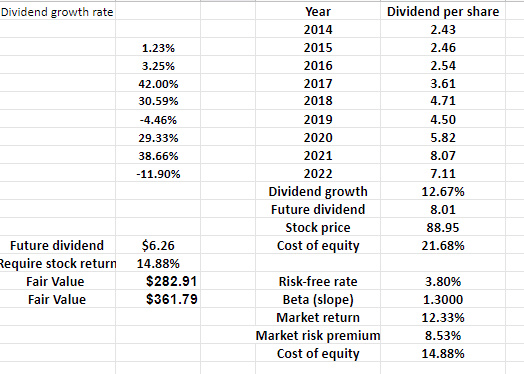# Morgan Stanley’s cost of equity, VaR, and theoretical stock price fair value

Nov 18, 2022Views: 375

Cost of Equity data series of Morgan Stanley At the minute, 3.8% risk-free rate, S&P500 market return=12.33% between 2014/22. Morgan Stanley’s future yearly dividend could be \$8.01. The CAPM cost of equity=14.88% seems less than the MSinternal cost of equity.

Variance CoVariance VaR Morgan Staley stock price volatility=1.97% 2014/22 data series. VaR Days 100, VarianceCovariance VaR= -3.18%.

very simple Gordon model, without checking the books. Possible to find the Dividend growth rate per year. With next year replicating a decline of -12% in dividends Morgan Stanley’s equity fair value= \$282.91 dollars. Assuming trend dividend growth of 12.67%, fair value=\$361.79 dollars.

continuous dividend discounted cashflows. Bear in mind that Required Stocks Returns= 14.88% with this Risk free rate=3.8%<4.0%